# Digital Image Processing Algorithms using MATLAB

• Difficulty Level : Hard
• Last Updated : 23 Feb, 2021

Like it is said, “One picture is worth more than ten thousand words “A digital image is composed of thousands and thousands of pixels.

An image could also be defined as a two-dimensional function, f(x, y), where x and y are spatial (plane) coordinates and therefore the amplitude of f at any pair of coordinates (x, y) is named the intensity or grey level of the image at that time. When x, y, and therefore the amplitude values of f are all finite, discrete quantities, we call the image a digital image.

## Image segmentation using various techniques

Following filters are used for Edge Detection and discontinuities of an image.

First derivative Operators:

• Sobel Mask– It is also used to detect two kinds of edges in an image one in Vertical and the other in Horizontal direction.
• Prewitt Mask– It is also used to detect two types of edges in an image, Horizontal and Vertical Edges. Edges are calculated by using the difference between corresponding pixel intensities of an image.
• Robert Mask– It is used in edge detection by approximating the gradient of an image through discrete differentiation.

Second derivative Operators:

• Laplacian– It is used to find areas of rapid change (edges) in images.
• LOG (Laplacian of a Gaussian) Mask (σ=3)- Since derivative filters are very sensitive to noise, it is common to smoothen the image (using a Gaussian filter) before applying the Laplacian. This two-step process is called the Laplacian of Gaussian (LoG) operation.
• Canny edge detector– It is a popular edge detection algorithm which is a multi-stage and gives the best results when compared to other algorithms.

The gradient-based method such as Prewitt has the most important drawback of being sensitive to noise. The Canny edge detector is less sensitive to noise but more expensive than Robert, Sobel and Prewitt. However, Canny edge detector performs better than all masks.

Input:Original Image

Code:

## MATLAB

 `clc;``close all;``clear all;`` ` `% Read Colour Image and convert it to a grey level Image``% Display the original Image``mycolourimage = imread(``'image1.jpg'``);``myimage = rgb2gray(mycolourimage);``subplot(3,3,1); ``imshow(myimage); title(``'Original Image'``);`` ` `% Apply Sobel Operator``% Display only the horizontal Edges``sobelhz = edge(myimage,``'sobel'``,``'horizontal'``);``subplot(3,3,2); ``imshow(sobelhz,[]); title(``'Sobel - Horizontal Edges'``);`` ` `% Apply Sobel Operator``% Display only the vertical Edges``sobelvrt = edge(myimage,``'sobel'``,``'vertical'``);``subplot(3,3,3); ``imshow(sobelhz,[]); title(``'Sobel - Vertical Edges'``);`` ` `% Apply Sobel Operator``% Display both horizontal and vertical Edges``sobelvrthz = edge(myimage,``'sobel'``,``'both'``);``subplot(3,3,4); ``imshow(sobelvrthz,[]); title(``'Sobel - All edges'``);`

Call the above function using the MATLAB command window.

Output: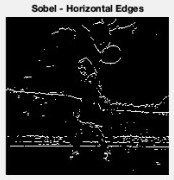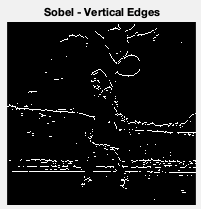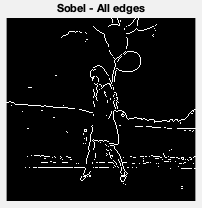Input:Original Image

Code:

## MATLAB

 `clc;``close all;``clear all;`` ` `% Read Colour Image and convert it to a grey level Image``% Display the original Image``mycolourimage = imread(``'image1.jpg'``);``myimage = rgb2gray(mycolourimage);``subplot(3,3,1); ``imshow(myimage); title(``'Original Image'``);`` ` `% Apply Prewitt Operator``% Display both horizontal and vertical Edges``Prewittsedg = edge(myimage,``'prewitt'``);``subplot(3,3,6); ``imshow(Prewittsedg,[]); title(``'Prewitt - Edges'``);`

Call the above function using the MATLAB command window.

Output: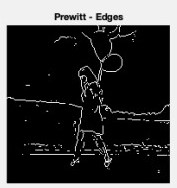Input:Original Image

Code:

## MATLAB

 `clc;``close all;``clear all;`` ` `% Read Colour Image and convert it to a grey level Image``% Display the original Image``mycolourimage = imread(``'image1.jpg'``);``myimage = rgb2gray(mycolourimage);``subplot(3,3,1); ``imshow(myimage); title(``'Original Image'``);`` ` `% Apply Roberts Operator``% Display both horizontal and vertical Edges``robertsedg = edge(myimage,``'roberts'``);``subplot(3,3,5); ``imshow(robertsedg,[]); title(``'Roberts - Edges'``);`

Call the above function using the MATLAB command window.

Output: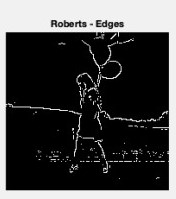Input:Original Image

Code:

## MATLAB

 `clc;``close all;``clear all;`` ` `% Read Colour Image and convert it to a grey level Image``% Display the original Image``mycolourimage = imread(``'image1.jpg'``);``myimage = rgb2gray(mycolourimage);``subplot(3,3,1); ``imshow(myimage); title(``'Original Image'``);`` ` `% Apply Laplacian Filter   ``f=fspecial(``'laplacian'``); ``lapedg = imfilter(myimage,f,``'symmetric'``); ``subplot(3,3,7); ``imshow(lapedg,[]); title(``'Laplacian Filter'``);`

Call the above function using the MATLAB command window.

Output: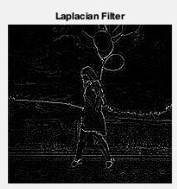Input:Original Image

Code:

## MATLAB

 `clc;``close all;``clear all;`` ` `% Read Colour Image and convert it to a grey level Image``% Display the original Image``mycolourimage = imread(``'image1.jpg'``);``myimage = rgb2gray(mycolourimage);``subplot(3,3,1); ``imshow(myimage); title(``'Original Image'``);`` ` `% Apply LOG edge detection  ``% The sigma used is 3 ``f=fspecial(``'log'``,[15,15],3.0);``logedg1 = edge(myimage,``'zerocross'``,[],f);``subplot(3,3,8); ``imshow(logedg1); title(``'Log with sigma 3'``);`

Call the above function using the MATLAB command window.

Output: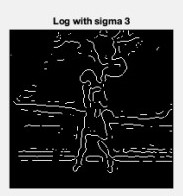#### Applying the Canny edge detector:

Input:Original Image

Code:

## MATLAB

 `clc;``close all;``clear all;`` ` `% Read Colour Image and convert it to a grey level Image``% Display the original Image``mycolourimage = imread(``'image1.jpg'``);``myimage = rgb2gray(mycolourimage);``subplot(3,3,1); ``imshow(myimage); title(``'Original Image'``);`` ` `% Apply Canny edge detection   ``cannyedg = edge(myimage,``'canny'``);``subplot(3,3,9); ``imshow(cannyedg,[]); title(``'Canny Edge'``);`

Call the above function using the MATLAB command window.

Output: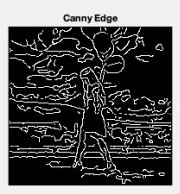Canny Edge Detector gives the best results compared to other filters/masks used to detect the edges in an image.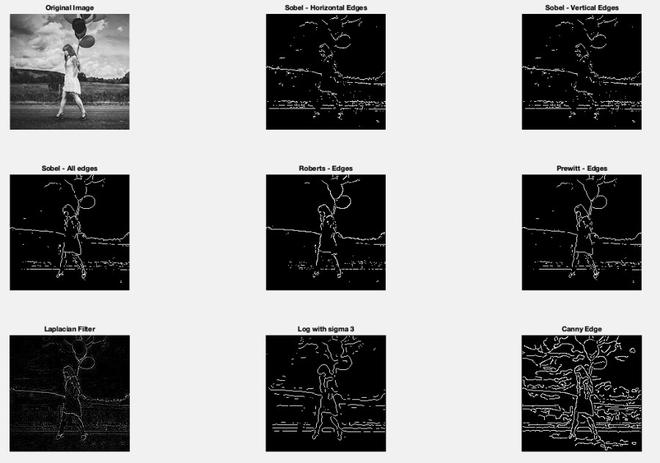Comparison of all filters used for Edge Detection

#### 2. Circular Hough Transform:

It is global processing and specialization of Hough Transform. It is used to detect the circles in an input image. This transform is selective to circles and ignores elongated ellipses.

Input: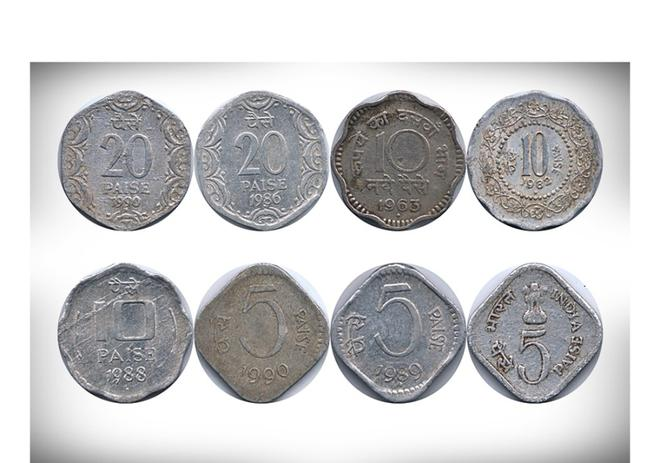Original Image

Code:

## MATLAB

 `clc;``close all;``clear all;`` ` `% Read Colour Image and convert it to a grey level Image``% Display the original Image``% Read the image that have circles``i=imread(``'image14.jpg'``);`` ` `% show image``imshow(i)`` ` `% select max & min threshold of circles we want to detect``Rmin = 10``Rmax = 50;`` ` `% Apply Hough circular transform``[centersDark1, radiiDark1] = imfindcircles(i, [Rmin Rmax],``'ObjectPolarity'``,``'dark'``,``'Sensitivity'``,0.92);`` ` `% show the detected circles by Red color --``viscircles(centersDark1, radiiDark1,``'LineStyle'``,``'--'``)`

Call the above function using the MATLAB command window.

Output: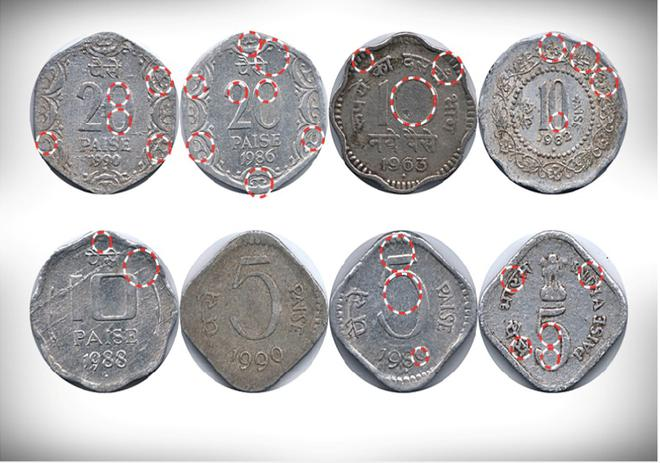Circular Hough Transformed Image

#### 3. Threshold Selection:

It is a local thresholding method in which we are thresholding an input image locally, passing some parameters. We took an image which is already edge operated.

Input:Original Image

Code:

## MATLAB

 `clc;``close all;``clear all;`` ` `% Read Colour Image and convert it to a grey level Image``% Display the original Image``image = imread(``'image2.jpeg'``);`` ` `mean_image = imfilter(image, fspecial(``'average'``,[15,15]),``'replica'``);``subtract = image - (mean_image+20);``black_white = im2bw(subtract,0);``subplot(1,2,1); imshow(black_white); title(``'Threshold Image'``);``subplot(1,2,2); imshow(image); title(``'Original Image'``);`

Call the above function using the MATLAB command window.

Output: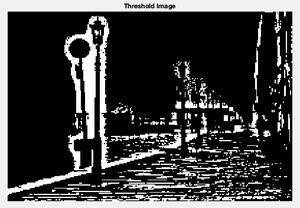#### 4. Otsu Method:

It is a method of optimum global thresholding. Otsu gives the best result when compared to threshold selection under local thresholding. This method minimizes the weighted within-class variance.

Input:Original Image

Code:

## MATLAB

 `clc;``close all;``clear all;`` ` `% Read Colour Image and convert it to a grey level Image``% Display the original Image``I1=imread(``'image2.jpeg'``);`` ` `%I1=rgb2gray(I);``imshow(I1);``figure, imhist(I1);``T2 = graythresh(I1);``it2= im2bw(I1,T2);``figure,imshow(it2);`

Call the above function using the MATLAB command window.

Output: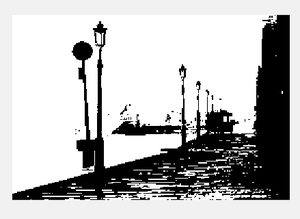Global Threshold Image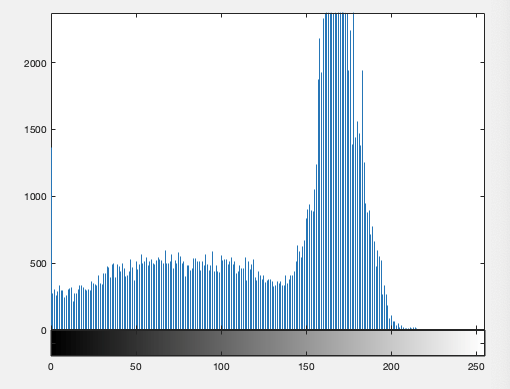Image Histogram

#### 5. Morphological watershed:

In this, the local minima of grey levels give the catchment basins and the local maxima define the watershed lines. In the output image, it is easy to detect the markers.

Input: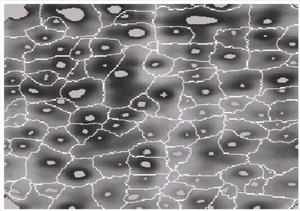Original Image

Code:

## MATLAB

 `clc;``close all;``clear all;`` ` `% Read Colour Image and convert it to a grey level Image``% Display the original Image``I= imread(``'image10.png'``);`` ` `%I rgb2gray(RGB);``I1 = imtophat(I, strel(``'disk'``, 10));``figure, imshow(I1);``I2 = imadjust(I1);``figure,imshow(I2);``level = graythresh(I2);``BW = im2bw(I2,level);``figure,imshow(BW);``C=~BW;``figure,imshow(C);``D = ~bwdist(C);``D(C) = -Inf;``L = watershed(D);``Wi=label2rgb(L,``'hot'``,``'w'``);``figure,imshow(Wi);``im=I;`

Call the above function using the MATLAB command window.

Output: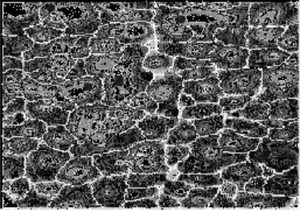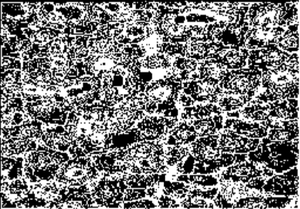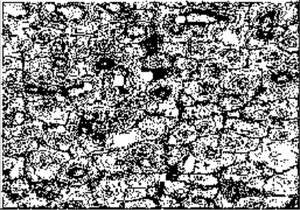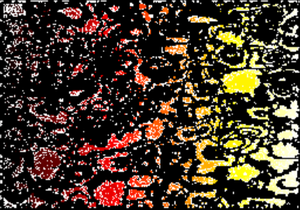#### 6. K-means Clustering:

It is an algorithm which is used to segment the interest area from the background. Partition the data points into K clusters randomly. Find the centroids of each cluster.

It operates on a 2D array where pixels in rows and RGB in columns. We take mean values for each class (K=3).

Input: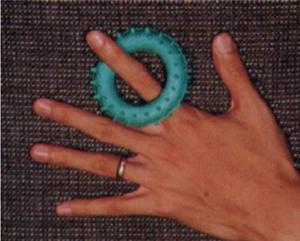Original Image

Code:

## MATLAB

 `clc;``close all;``clear all;`` ` `% Read Colour Image and convert it to a grey level Image`` ` `% Load in an input image``im = imread(``'image12.png'``);`` ` `% We also cast to a double array, because K-means requires it in matlab``imflat = double(reshape(im, size(im,1) * size(im,2), 3));``K = 3``[kIDs, kC] = kmeans(imflat, K, ``'Display'``, ``'iter'``, ``'MaxIter'``, 150, ``'Start'``, ``'sample'``);``colormap = kC / 256;`` ` `% Scale 0-1, since this is what matlab wants``% Reshape kIDs back into the original image shape``imout = reshape(uint8(kIDs), size(im,1), size(im,2));``imwrite(imout - 1, colormap, ``'image6.jpg'``);`

Call the above function using the MATLAB command window.

Output:

```K =
3
iter  phase      num          sum
1      1   178888  1.52491e+08
2      1     7657  1.45223e+08
3      1     4597  1.42317e+08
4      1     3750  1.40017e+08
5      1     3034  1.38203e+08
6      1     2187  1.37096e+08
7      1     1552  1.36481e+08
8      1     1044  1.36165e+08
9      1      701  1.36014e+08
10      1      479  1.35939e+08
11      1      311  1.35906e+08
12      1      282  1.35883e+08
13      1      193   1.3587e+08
14      1      124  1.35865e+08
15      1       85  1.35863e+08
16      1       60  1.35861e+08
17      1       80   1.3586e+08
18      1       79  1.35858e+08
19      1       23  1.35858e+08
20      1       48  1.35858e+08
21      1        7  1.35858e+08
Best total sum of distances = 1.35858e+08```

My Personal Notes arrow_drop_up